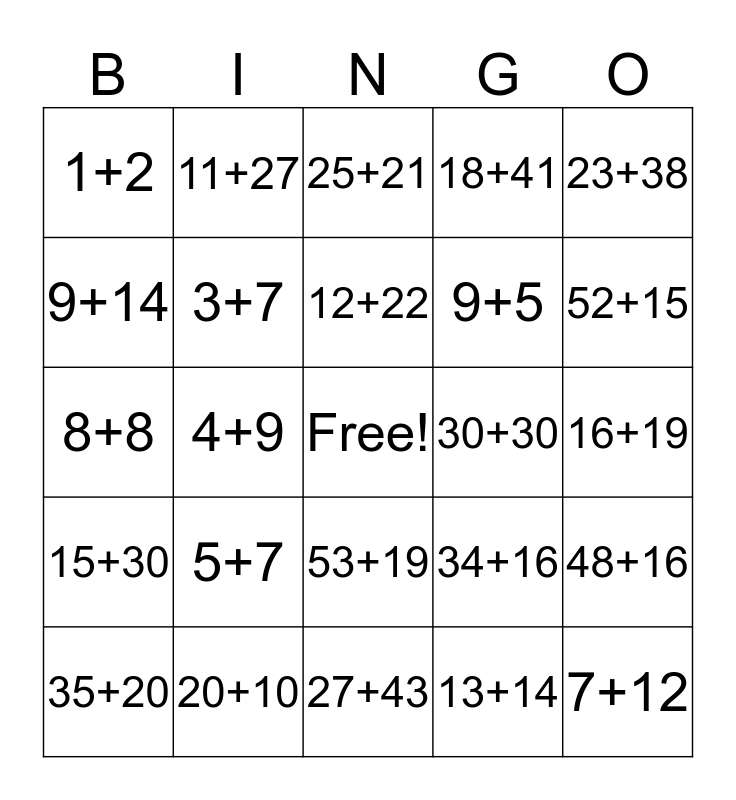This bingo card has a free space and 24 words: 5+7, 20+10, 12+22, 35+20, 23+38, 1+2, 9+14, 11+27, 30+30, 53+19, 9+5, 8+8, 34+16, 48+16, 3+7, 13+14, 16+19, 25+21, 52+15, 4+9, 7+12, 15+30, 18+41 and 27+43.

## Play Online